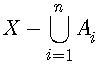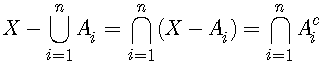## The Inclusion-Exclusion Principle

From the First Principle of Counting we have arrived at the commutativity of addition, which was expressed in convenient mathematical notations as a + b = b + a. The Principle itself can also be expressed in a concise form. It consists of two parts. The first just states that counting makes sense. The second describes its fundamental property.

1. Every group of objects A can be associated with a quantity - denoted |A| - called the number of elements in A.
2. If X = AB and AB = Ø, then |X| = |A| + |B|.

The latter is a statement of legitimacy of counting by grouping. If a group of objects X is split into two groups - denoted A and B, which means that they have no common elements (AB = Ø) and together combine into the whole (X = AB), then the number of elements |X| in the group X can be arrived at by first counting elements of A and then counting elements of B. We shall consider that statement on its own merits.

Since X = AB, the idea of counting by grouping can also be expressed as

 (1) If A∩B = Ø, then |A∪B| = |A| + |B|.

This is exactly same statement with a somewhat different emphasis. Instead of splitting the whole into two groups, we start with two (disjoint, i.e. with no common elements) wholes and combine them into one. The latter form is suggestive of the question, What if A and B are not disjoint? Or is it?

Is it not too artificial to count sets that are not disjoint? After all, this would never happen with counting by grouping. Well, what can one say? Is it not what is called Turning an idea around in one's mind? Once the humankind began composing the story of counting, the plot acquired life and logic of its own. and this is how - by following its plot - we arrived at where we are today: masters of a vast amount of accumulated knowledge in control of fantastically powerful technology that could not have been foreseen at the beginning of the story. (Consider also such a question: two brothers have three siblings each. How many children are in the family?)

If A and B are not disjoint, we get the simplest form of the Inclusion-Exclusion Principle:

 (2) |A∪B| = |A| + |B| - |A∩B|.

Indeed, in |A| + |B| some elements have been counted twice (the common siblings of the two brothers). Which are they? The elements that were counted twice are exactly those that belong to A (one count) and also belong to B (the second count). In short, counted twice were the elements of AB. To obtain an accurate number |AB| of elements in the union we have to subtract from |A| + |B| the number |AB| of such elements.

Here's an argument that may appear more rigorous.

Since AB and B - A are disjoint as are A and B - A, and moreover AB = A∪(B - A) and B = (AB)∪(B - A), it follows from (1) that

 (3) |A∪B| = |A| + |B - A| |A∩B| + |B - A| = |B|,

The story of course does not end here. What about if there are three sets: A, B, C? For three sets, the Inclusion-Exclusion Principle reads

 (2') |A∪B∪C| = |A| + |B| + |C| - |A∩B| - |B∩C| - |A∩C| + |A∩B∩C|

We could derive (2') from (2) in the manner of (3) - and this is a good exercise in using set-theoretical notations. But there is another approach with a more manageable generalization to the case of any finite number of sets, not just three. (You did not think we'd stop at (2')?)

Let x be an element of ABC. As such, it's counted once in the left-hand side of (2'). x may belong to 1, 2, or 3 sets A, B, C. Assume it belongs only to A. Then on the right of (2') it is counted only once in |A|.

Let x belong to A and B. On the right, it is counted in |A|, |B|, and |AB| - twice added, once subtracted.

Lastly, let x belong to all three sets A, B, C. It is then counted in every term of (2') - 4 times added and 3 times subtracted - again adding up to 1.

In the more general case where there are n different sets Ai, the formula for the Inclusion-Exclusion Principle becomes:

 (4)What does (4) say? On the left is number of elements in the union of n sets. On the right, we first count elements in each of the sets separately and add the up. as we already know, if the sets Ai are not disjoint, some elements will have be counted more than once. Those are the elements that belong to at least two of the sets Ai, or the intersections AiAj. We wish to consider every such intersection, but each only once. Since AiAj = AjAi, to avoid duplications we arbitrarily decide to consider only pairs (Ai, Aj) with i < j.

When we subtract the sum of the number of elements in such pairwise intersections, some elements may have been subtracted more than once. Those are the elements that belong to at least three of the sets Ai. We add the sum of the elements of intersections of the sets taken three at a time. (The condition i < j < k assures that every intersection is counted only once.)

The process goes on with sums being alternately added or subtracted until we come to the last term - the intersection of all sets Ai. Whether it's added or subtracted depends on n: for n = 2 it was subtracted, for n = 3 added - take a clue from here.

How does one prove (4)? Assume that an element x ofbelongs to exactly r of the sets Ai. If that's the case, all intersections of more than r sets are empty - contain no elements. Moreover, all intersections in which one of sets does not contain x are also empty. Of the given r sets that do contain x, we may form C(r, 2) = r(r - 1)/2 2-set intersections, C(r, 3) = r(r - 1)(r - 2)/3! 3-set intersections, and so on. Each of those intersections will of course be counted only once in (4), which thus reduces to

 1 = C(r, 1) - C(r, 2) + C(r, 3) - .... + (-1)r-1C(r, r)

or,

 1 - C(r, 1) + C(r, 2) - C(r, 3) + .... + (-1)rC(r, r) = (1 - 1)r = 0

by the binomial theorem. This completes the proof of (4).Sets Ai are often taken to be subsets of a larger set X such that each Ai is a collection of elements of X that share some property Pi.is then the subset of X that consists of all elements of X having at least one of the properties Pi. Its complementis the set of elements that have none of those properties:from which

 || = | X | - ||.

 (4')The left-hand side in (4') gives the number of elements of X that have none of the properties Pi.Let X be the set of all permutations of m elements. | X | = m! Let Ai be the set of all permutations that leave the i-the element of X fixed. Obviously, |Ai| = (m - 1)!. The set AiAj leaves two elements (i-th and j-th) fixed. |AiAj| = (m - 2)! There are C(m, 2) such pairwise intersections. Continuing along this path leads to the formulaOn the left, we got the number of derangements - permutations that move every single element - whose set is often denoted as Dm:

 (5) |Dm| = m! - m·(m - 1)! + C(m, 2)(m - 2)! - ... + (-1)mC(m, m) = m! (1 - 1/1! + 1/2! - 1/3! + ... + (-1)m / m!).

We have encountered derangements while labeling boxes. D3 = 2 and this is why the puzzle was easy. D4 = 9, which is why the generalization of the puzzle was hard.

### References

1. V. K. Balakrishnan, Theory and Problems of Combinatorics, Schaum's Outline Series, McGraw-Hill, 1995
2. J. Havil, Nonplussed!, Princeton University Press, 2007
3. S. Lipschutz and M. L. Lipson, 2000 Solved Problems in Discrete Mathematics, McGraw-Hill, 1992• The Basic Rules of Counting
• Combinatorial Proofs
• Fibonacci Tilings
• Inclusion-Exclusion Principle: an Example
• Ramsey's Theorem
• Coloring Points in the Plane
• Probabilities
• Example: A Poker Hand
•